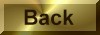Practice Problem 3

Use the rate constant for the reaction between phenolphthalein and the OH- ion to calculate the initial instantaneous rate of reaction for the experimental data listed in the preceding table.

Solution

Substituting the rate constant for the reaction from the preceding problem and the initial concentration of phenolphthalein into the rate law for this reaction gives the following:

Rate = k(phenolphthalein) = (0.010 s-1)(0.0050 mol/L) = 5.0 x 10-5 (mol/L)/s

Because the rate of reaction is the change in the concentration of phenolphthalein divided by the time over which the change occurs, it is reported in units of moles per liter per second.  Because the number of moles of phenolphthalein per liter is the molarity of this solution, the rate can also be reported in terms of the change in molarity per second: M/s.